# Work, Power, Potential Energy and Kinetic Energy Relations (Part - 5) Civil Engineering (CE) Notes | EduRev

## Civil Engineering (CE) : Work, Power, Potential Energy and Kinetic Energy Relations (Part - 5) Civil Engineering (CE) Notes | EduRev

The document Work, Power, Potential Energy and Kinetic Energy Relations (Part - 5) Civil Engineering (CE) Notes | EduRev is a part of the Civil Engineering (CE) Course Introduction to Dynamics and Vibrations- Notes, Videos, MCQs.
All you need of Civil Engineering (CE) at this link: Civil Engineering (CE)

4.1.12 Power transmission in machines

A machine is a system that converts one form of motion into another. A lever is a simple example – if point A on the lever is made to move, then point B will move either faster or slower than A, depending on the lengths of the two lever arms. Other, more practical examples include

1. Your skeleton; used to help convert muscle motion into various more useful forms…
2. The transmission of a vehicle – used to convert rotational motion induced by the engine’s crank-shaft to translational motion of the vehicle
3. A gearbox – converts rotational motion at one angular speed to rotational motion at another.
4. A system of pulleys.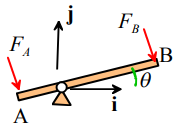Concepts of work and energy are extremely useful to analyze transmission of forces and moments through a machine. The goal of these calculations is to quickly determine relationships between external forces acting on the machine, rather than to analyze the motion of the system itself.

To do this, we usually make two assumptions:

1. The machine is a conservative system. This is true as long as friction in the machine can be neglected.
2. The kinetic and potential energy of the mechanism within the machine can be neglected. This is true if either (i) the machine has negligible mass and is very stiff (i.e. it does not deform); or (ii) the machine moves at steady speed or is stationary.

If this is the case, the total rate of work done by external forces acting on the machine is zero. This principle can be used to relate external forces acting on the machine, without having to work through the very lengthy and tedious process of computing all the internal forces.

The procedure to do this is always

1. Derive a relationship between the velocities of the points where external forces act – this is always a geometric relationship.
2. Write down the total rate of external work done on the system
3. Use (1) and (2) to relate the external forces to each other.
The procedure is illustrated using a number of examples below.

Example 1: As a first example, we derive a formula relating the forces acting on the two ends of a lever. Assume that the forces act perpendicular to the lever as shown in the figure.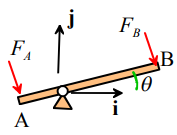Calculation

1. Suppose that the lever rotates about the pivot at some angular rate dθ / dt = ω.
2. The ends of the lever have velocities vA = ωdB (sinθi - cosθi + cosθj) (see the circular motion example in the preceding chapter if you can’t remember how to show this.
3. The force vectors can be expressed as FA = FA(sinθi - cosθj) FB = FB (sinθi - cosθj)
4. The reaction forces at the pivot are stationary and so do no work.
5. The total rate of work done on the lever is therefore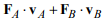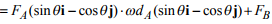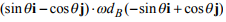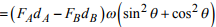6. The rate of external work is zero, so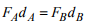Example 2: Here we revisit an EN3 statics problem. The rabbit uses a pulley system to raise carrot with weight W. Calculate the force applied by the rabbit on the cable.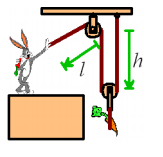1. The velocity of the end of the cable held by the rabbit is dl/dt, with direction parallel to the cable

2. The velocity of the carrot is dh/dt ; its direction is vertical
3. The total length of the cable (aside from small, constant lengths going around the pulleys) is l +2h . Since the length is constant, it follows that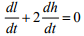4. The external forces acting on the pulley system are (i) the weight of the carrot W, acting vertically; (ii) the unknown force F applied by the rabbit, acting parallel to the cable; and (iii) the reaction force acting on the topmost pulley. The rate of work done by the rabbit is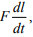the rate of work done by the gravitational force acting on the carrot is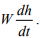The reaction force is stationary, and so does no work. The total rate of work done is therefore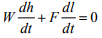5. Using (3) and (4) we see that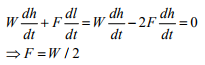Example 3 Calculate the torque that must be applied to a screw with pitch d in order to raise a weight W.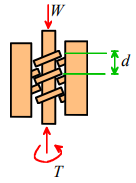Here, we regard the screw as a machine subjected to external forces.
1. When the screw turns through one complete turn (2π radians) it raises the weight by a distance d
2. The work done by the torque is 2πT ; the work done by the weight is – Wd. The reaction forces acting on the thread of the screw do no work
3. The total work is zero, therefore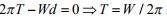Example 4: A gearbox is used for two purposes: (i) it can amplify or attenuate torques, or moments; and (ii) it can change the speed of a rotating shaft. The characteristics of a gearbox are specified by its gear ratio specifies the ratio of the rotational speed of the output shaft to that of the input shaft r = ω0 / ωi.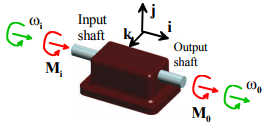The sketch shows an example. A power source (a rotational motor) drives the input shaft; while the output shaft is used to drive a load. The rotational motion of the input and output shafts can be described by angular velocity vectors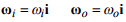(note that the shafts don’t necessarily have to rotate in the same direction – if they don’t then one of  ω0 or ωi  will be negative).

A quick power calculation can be used to relate the moments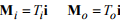acting on the input and output shafts.

1. The gear ratio relates the input and output angular velocities as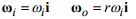2. The rate of work done on the gearbox by the moment acting on the input shaft is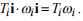The work done by the moment acting on the output shaft is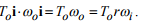A reaction moment must act on the base of the gearbox, but since the gearbox is stationary this reaction moment does no work.

3. The rate of work done by all the external forces on the gearbox is zero, so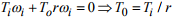Example 5: Find a formula for the engine power required for a vehicle with mass m to climb a slope with angle α at speed v. Estimate values for engine power required for various vehicles to climb a slopes of 3%, 4% and 5% (these are typical standard grades for flat, rolling and mountain terrain freeways, see, e.g.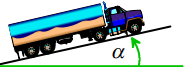Also, estimate (a) the torque exerted by the engine crankshaft on the transmission; and (b) the force acting on the pistons of the engine.

Assumptions:

1. The only forces acting on the vehicle are aerodynamic drag, gravity, and reaction forces at the driving wheels.
2. Aerodynamic drag will be assumed to be in the high Reynolds number regime, with a drag coefficient of order
3. The crankshaft rotates at approximately 2000rpm.
4. As a representative examples of engines, we will consider (i) a large 6 cylinder 4 stroke diesel engine, with stroke (distance moved by the pistons) of 150mm; and (ii) a small 4 cylinder gasoline powered engine with stroke 94mm

Calculation. The figure shows a free body diagram for the truck. It shows (i) gravity; (ii) Aerodynamic drag; (iii) reaction forces acting on the wheels.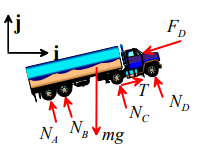1. The velocity vector of the vehicle is  v(cosαi + sinαj)
2. The rate of work done by the gravitational and aerodynamic forces is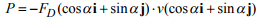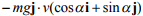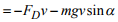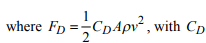the drag coefficient, A the frontal area of the vehicle, and p the air density.
3. The reaction forces acting on the wheels do no work, because they act on stationary points (the wheel is not moving where it touches the ground).
4. The kinetic energy of the vehicle is constant, so the total rate of work done on the vehicle must be zero. The moving parts of the engine (specifically, the pressure acting on the pistons) do work on the vehicle. Denoting the power of the engine by PE , we have that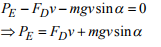5. The torque exerted by the crankshaft can be estimated by noting that the rate of work done by the engine is related to the torque T and the rotational speed ω of the crankshaft by PE = Tω. The torque follows as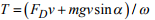6. The force F on the pistons can be estimated by noting that the rate of work done by a piston is related to the speed of the piston v by P = Fv. In a four-stroke engine, each piston does work 25% of the time (during the firing stroke). If the engine has n cylinders, the total engine power is therefore PE = nFv / 4 Finally, the piston speed can be estimated by noting that the piston travels the distance of the stroke during half a revolution, and so has average speed v = dω / π . This shows that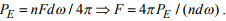The table below calculates values for two examples of vehicles

 Vehicle Grade Weight (kg) Frontal aream2 Drag Coeft Speedm/s Engine speedRad/sec Strokem Engine power Watts TorqueNm Piston forceN 18wheeler 3% 36000 10 0.1 29 12600 0.15 320000 25 355 4% 420000 34 470 5% 520000 42 580 Chevy 3% 14000 1.1 36 cobalt 4% 1250 2.5 0.1 29 12600 0.095 17000 1.4 46 5% 21000 1.7 55
Offer running on EduRev: Apply code STAYHOME200 to get INR 200 off on our premium plan EduRev Infinity!

## Introduction to Dynamics and Vibrations- Notes, Videos, MCQs

20 videos|53 docs

,

,

,

,

,

,

,

,

,

,

,

,

,

,

,

,

,

,

,

,

,

,

,

,

,

,

,

;